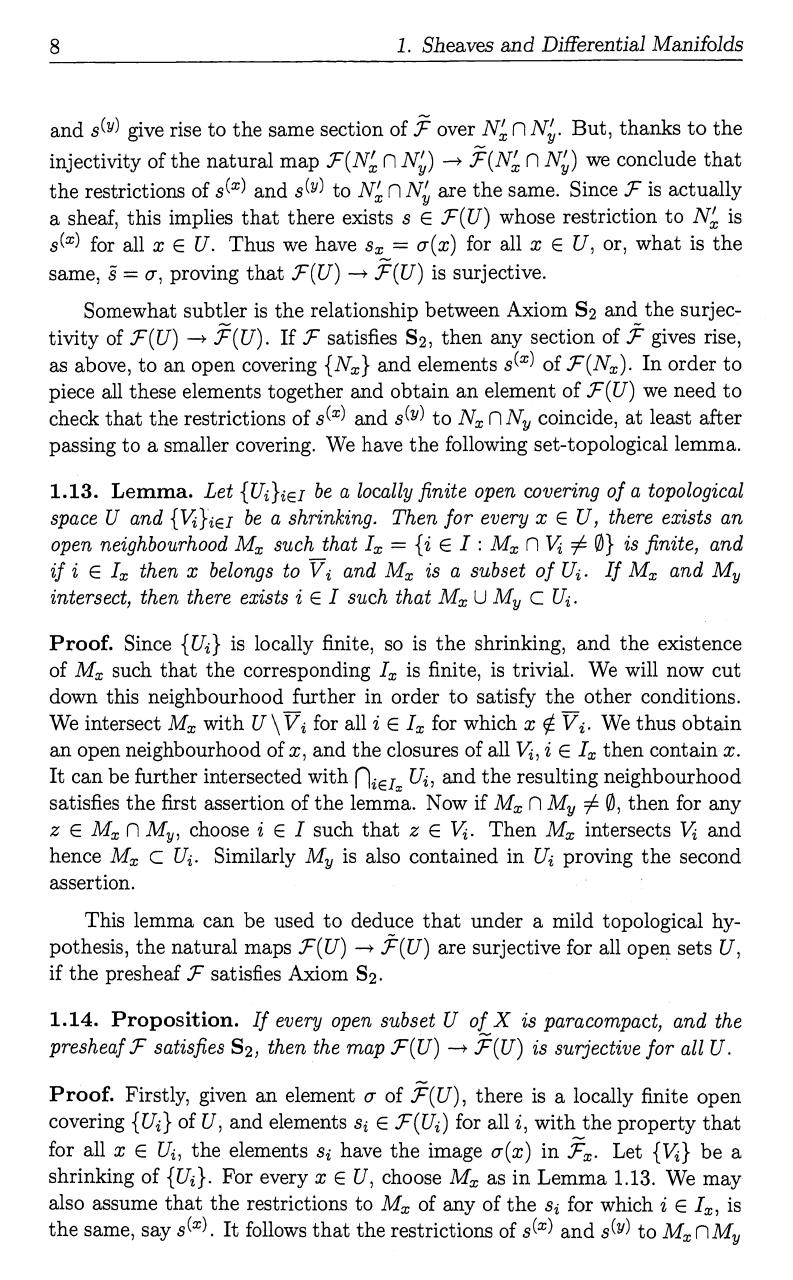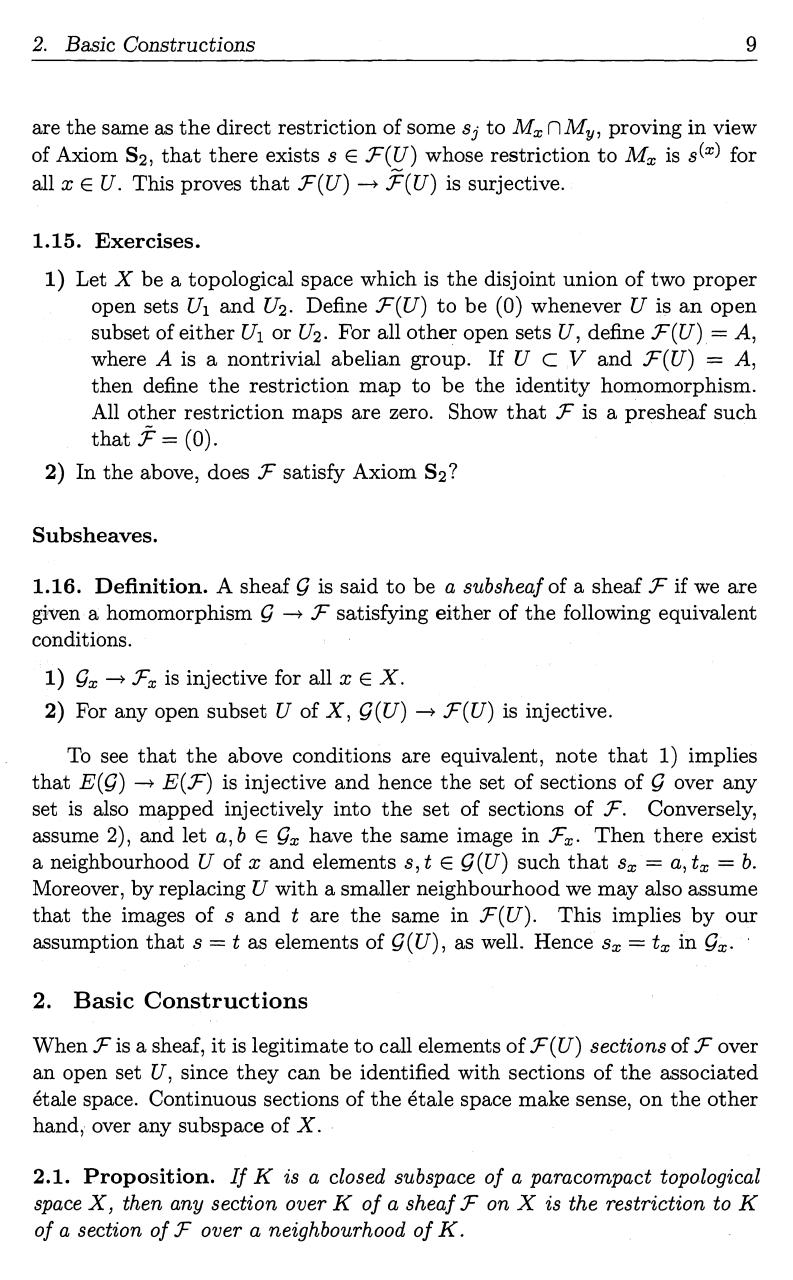# DiffGeoSheavesRamanan.21 22 .pdf

### File information

Original filename: DiffGeoSheavesRamanan.21-22.pdf

This PDF 1.4 document has been generated by / iTextÂ® 5.5.8 Â©2000-2015 iText Group NV (ONLINE PDF SERVICES; licensed version), and has been sent on pdf-archive.com on 13/06/2016 at 00:12, from IP address 69.250.x.x. The current document download page has been viewed 388 times.
File size: 51 KB (2 pages).
Privacy: public file

DiffGeoSheavesRamanan.21-22.pdf (PDF, 51 KB)

### Document preview

8

1. Sheaves and Differential Manifolds

and s() give rise to the same section of J over N n N. But, thanks to the
injectivity of the natural map .F(N, n N,) -j :'(N,,' n N,) we conclude that
the restrictions of s(x) and s(Y) to NN n Ny are the same. Since F is actually
a sheaf, this implies that there exists s E .7= (U) whose restriction to Nx is

slxl for all x E U. Thus we have sx= u(x) for all x c U, or, what is the
same, s = or, proving that F(U) , F(U) is surjective.
Somewhat subtler is the relationship between Axiom S2 and the surjecIf F satisfies S2, then any section of F gives rise,
as above, to an open covering {Nx} and elements s(x) of 1F(Nx). In order to
piece all these elements together and obtain an element of F(U) we need to
check that the restrictions of s(x) and s(y) to Nx n N. coincide, at least after
passing to a smaller covering. We have the following set-topological lemma.

tivity of F(U) -*

1.13. Lemma. Let {Ui}iEI be a locally finite open covering of a topological
space U and {V }iEI be a shrinking. Then for every x E U, there exists an
open neighbourhood Mx such that Ix = {i E I : Mx n V O} is finite, and

if i E Ix then x belongs to Vi and Mx is a subset of Ui. If Mx and M.
intersect, then there exists i E I such that Mx U My C U.

Proof. Since {Ui} is locally finite, so is the shrinking, and the existence
of M. such that the corresponding Ix is finite, is trivial. We will now cut
down this neighbourhood further in order to satisfy the other conditions.
We intersect Mx with U \Vi for all i E Ix for which x 0 V2. We thus obtain
an open neighbourhood of x, and the closures of all V, i E Ix then contain x.
It can be further intersected with niElx Ui, and the resulting neighbourhood
satisfies the first assertion of the lemma. Now if Mx n My 0 0, then for any

z E Mx n My, choose i E I such that z E V. Then Mx intersects V and
hence Mx C Ui. Similarly My is also contained in Ui proving the second
assertion.

This lemma can be used to deduce that under a mild topological hypothesis, the natural maps .F(U) -+ F(U) are surjective for all open sets U,
if the presheaf F satisfies Axiom S2.

1.14. Proposition. If every open subset U of X is paracompact, and the
presheaf _'F satisfies S2, then the map F(U) -+ F(U) is surjective for all U.

Proof. Firstly, given an element o- of F(U), there is a locally finite open
covering {Ui} of U, and elements si E F(Ui) for all i, with the property that
for all x E Ui, the elements si have the image o-(x) in F. Let {V } be a
shrinking of {Ui}. For every x E U, choose M. as in Lemma 1.13. We may
also assume that the restrictions to Mx of any of the si for which i E Ix, is
the same, say s(x). It follows that the restrictions of s(x) and s(') to MxnMy

2.

Basic Constructions

9

are the same as the direct restriction of some si to m, fl my, proving in view
of Axiom S2, that there exists s E F(U) whose restriction to Mx is s(x) for
all x E U. This proves that .r(U) --&gt; F(U) is surjective.

1.15. Exercises.
1) Let X be a topological space which is the disjoint union of two proper
open sets U1 and U2. Define .F(U) to be (0) whenever U is an open
subset of either U1 or U2. For all other open sets U, define .F(U) = A,
where A is a nontrivial abelian group. If U C V and F(U) = A,
then define the restriction map to be the identity homomorphism.
All other restriction maps are zero. Show that F is a presheaf such
that .,' = (0).
2) In the above, does F satisfy Axiom S2?

Subsheaves.
1.16. Definition. A sheaf Q is said to be a subsheaf of a sheaf F if we are
given a homomorphism -&gt; F satisfying either of the following equivalent
conditions.

Fx is injective for all x E X.
2) For any open subset U of X, Q(U) -&gt; F(U) is injective.
1) Qx

To see that the above conditions are equivalent, note that 1) implies
that E(Q) -+ E(F) is injective and hence the set of sections of Q over any
set is also mapped injectively into the set of sections of F. Conversely,
assume 2), and let a, b E Qx have the same image in F. Then there exist
a neighbourhood U of x and elements s, t E Q(U) such that sx = a, tx = b.
Moreover, by replacing U with a smaller neighbourhood we may also assume

that the images of s and t are the same in F(U). This implies by our
assumption that s = t as elements of Q(U), as well. Hence sx = tx in Q.
2.

Basic Constructions

When .F is a sheaf, it is legitimate to call elements of F(U) sections of F over

an open set U, since they can be identified with sections of the associated
etale space. Continuous sections of the etale space make sense, on the other
hand, over any subspace of X.

2.1. Proposition. If K is a closed subspace of a paracompact topological
space X, then any section over K of a sheaf F on X is the restriction to K
of a section of Jc' over a neighbourhood of K.#### HTML Code

Copy the following HTML code to share your document on a Website or Blog

#### QR Code### Related keywords